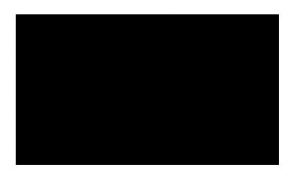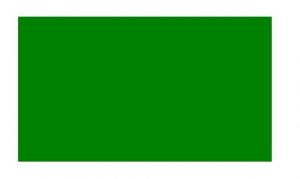Related Articles
HTML | canvas fillRect() Method
• Last Updated : 24 May, 2019

The fillRect() method is used to fill the rectangle using the given color. The default color of the fillRect() method is black.

Syntax:

`context.fillRect( x, y, width, height )`

Parameters: This method accepts four parameters as mentioned above and described below:

• x: It stores the x-coordinate of top-left corner of rectangle.
• y: It stores the y-coordinate of top-left corner of rectangle.
• width: It stores the width in pixel.
• height: It stores the height in pixel.

Example 1: This example uses fillRect() method to create rectangle and filled with default color (black).

 ```<``html``>``<``head``>``    ``<``title``>``        ``HTML canvas fillRect() Method``    ``````<``body``>``    ``<``canvas` `id``=``"GFG"` `width``=``"500"` `height``=``"300"``>``     ` `    ``<``script``>``        ``var x = document.getElementById("GFG");``        ``var contex = x.getContext("2d");``        ``contex.fillRect(50, 50, 350, 200);``        ``contex.stroke();``    `` `` ` ````

Output:Example 2: This example uses fillRect() method to create rectangle and filled with ve color (green).

 ```<``html``>``<``head``>``    ``<``title``>``        ``HTML canvas fillRect() Method``    ``````<``body``>``    ``<``canvas` `id``=``"GFG"` `width``=``"500"` `height``=``"300"``>``     ` `    ``<``script``>``        ``var x = document.getElementById("GFG");``        ``var contex = x.getContext("2d");``        ``contex.fillStyle = "green";``        ``contex.fillRect(50, 50, 350, 200);``        ``contex.stroke();``    `` `` ` ````

Output:Supported Browsers: The browsers supported by fillRect() method are listed below: